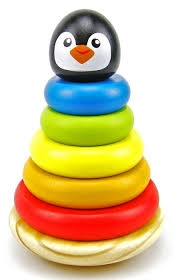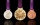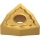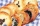# Toys 3

Tiffany's toyshop received a shipment of 360 toys. The first day 12 were sold the second day 19 were sold and on the third day, 26 was sold. How many days will the toyshop run out of toys?

Correct result:

n =  22

#### Solution:We would be pleased if you find an error in the word problem, spelling mistakes, or inaccuracies and send it to us. Thank you!Tips to related online calculators
Looking for help with calculating arithmetic mean?
Looking for a statistical calculator?
Do you want to round the number?

#### You need to know the following knowledge to solve this word math problem:

We encourage you to watch this tutorial video on this math problem:

## Next similar math problems:

• RewardJanko and Peter divided the reward from the brigade so that Peter got 5/8 of the reward. What percentage of Janko's reward got?
• Nineteenth memberFind the nineteenth member of the arithmetic sequence: a1=33 d=5 find a19
• Art schoolEvery fifth pupil 9A goes to art school. How many percent of pupils in class 9A go to art school?
• 5 peopleFive people have $122000 each, and one person has$539000. How much should each person (equally) pay?
• Three brothersThe three brothers have a total of 42 years. Jan is five years younger than Peter and Peter is 2 years younger than Michael. How many years has each of them?
• MushroomsEva and Jane collected 114 mushrooms together. Eve found twice as much as Jane. How many mushrooms found each of them?
• ScrewsThe box contains 8 iron, 6 brass, and 4 titanium screws. What is the probability that the randomly selected screw will not be brass?
• TreesA certain species of tree grows an average of 0.5 cm per week. Write an equation for the sequence that represents the weekly height of this tree in centimeters if the measurements begin when the tree is 200 centimeters tall.
• AverageIf the average(arithmetic mean) of three numbers x,y,z is 50. What is the average of there numbers (3x +10), (3y +10), (3z+10) ?
• Sequence 2Write the first 5 members of an arithmetic sequence a11=-14, d=-1
• Hotel roomsIn the 45 rooms, there were 169 guests, some rooms were three-bedrooms and some five-bedrooms. How many rooms were?
• Two numbersThe difference between the two numbers is 74. If we divide a larger number by a smaller one, we get a quotient 7 and the rest of 2. Determine both numbers.
• SequenceWrite the first 7 members of an arithmetic sequence: a1=-3, d=6.
• 6 termsFind the first six terms of the sequence. a1 = 7, an = an-1 + 6Montealegre family has a catering business. In one birthday party, they charged their costumer 11,767.50 for a party of 90 persons and a service charge of 2,500.50. How much was the average cost per person? Please show your solution.Theatrical performance was attended by 480 spectators. Women were in the audience 40 more than men and children 60 less than half of adult spectators. How many men, women and children attended a theater performance?Insert arithmetic means between 75 and 180.